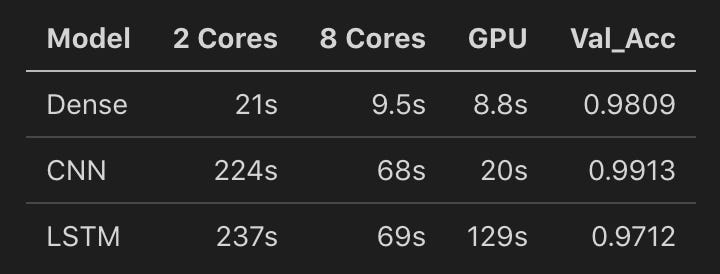# Compare TensorFlow performances on CPUs and GPUs

Source: Deep Learning on Medium

For many people Deep Learning means using GPU but is it always true in any situation and for any kind of network?

This study compares training performances of Dense, CNN and LSTM models on CPU and GPUs, by using TensorFlow high level API (Keras).

I use the same setup for every test running on Floydhub.

• CPU : 2 and 8 Cores Intel(R) Xeon(R) Platinum 8175M CPU @ 2.50GHz
• GPU : Tesla K80 12 GB RAM
• Python 3.6.5
• Tensorflow 1.13.1
• Dataset : MNIST
• Training Set Size : 60 000 images
• Epochs : 5
• Batch Size : 128

Here I use the standard TensorFlow instance as provided by Floydhub. This is important as this distribution doesn’t use the Intel MKL optimizations.

Using MNIST for a LSTM could sound strange, but this is something already explored by some authors with surprisingly not so bad results. Here we consider the 28×28 images as 28 time-steps of 28 features each.

The models tested are the following:

Dense

``model = tf.keras.models.Sequential()model.add(layers.Dense(512,activation='relu',input_shape=(28*28,)))model.add(layers.Dropout(rate=0.2))model.add(layers.Dense(64,activation='relu'))model.add(layers.Dense(10,activation='softmax'))model.compile(optimizer='rmsprop', loss='categorical_crossentropy', metrics=['accuracy'])``

CNN

``model = tf.keras.models.Sequential()model.add(layers.Conv2D(32,(3,3),activation = 'relu',input_shape=(28,28,1)))model.add(layers.MaxPooling2D((2,2)))model.add(layers.Conv2D(64,(3,3),activation = 'relu'))model.add(layers.MaxPooling2D((2,2)))model.add(layers.Conv2D(64,(3,3),activation = 'relu'))model.add(layers.Flatten())model.add(layers.Dense(64,activation='relu'))model.add(layers.Dense(10,activation='softmax'))model.compile(optimizer = 'rmsprop', loss = 'categorical_crossentropy', metrics = ['accuracy'])``

LSTM

``model = tf.keras.models.Sequential()model.add(layers.LSTM(128,input_shape=train_images.shape[1:]))model.add(layers.Dense(10,activation='softmax'))model.compile(optimizer = 'rmsprop', loss = 'categorical_crossentropy', metrics = ['accuracy'])``

#### Test ResultsTest Results for CPU, GPU and Validation set Accuracy

Clearly the benefits from the GPU is only significant for the CNN.

For Dense networks, the difference between the 8 Cores CPU and GPU is quite small and does not justify the investment in a GPU. Anyway, this needs to be tested again for very deep Dense networks.

The LSTM is the one taking the biggest benefits from the CPU power compared to the GPU with a training on 8 Xeon Cores nearly 1.9 times faster than with a Tesla K80 GPU.

So before training my networks, I simply use these rules as guideline:

• CNN : GPU most of the time, and especially for long training
• Dense : always train at first on a CPU with 4 cores, 8 Cores or more before to jump on a GPU. It could be much cheaper and much more flexible to use an instance with many CPU cores instead of a GPU
• LSTM : I never use GPU for RNN, just add CPU cores to improve training performances

I hope this short benchmark will help many other Data Scientists in their research process.

Thank you for reading.